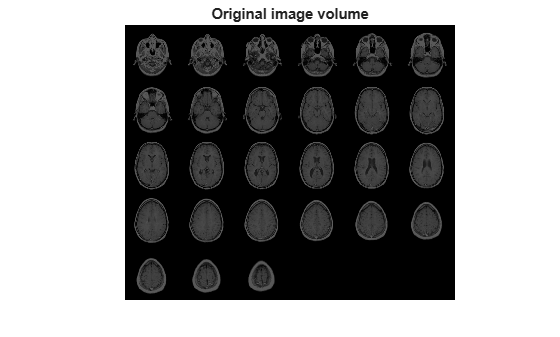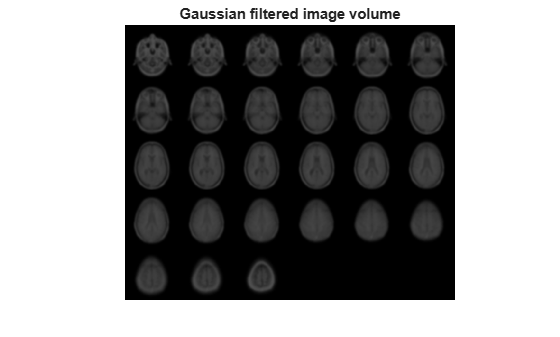# imgaussfilt3

3-D Gaussian filtering of 3-D images

## Syntax

``B = imgaussfilt3(A)``
``B = imgaussfilt3(A,sigma)``
``B = imgaussfilt3(___,Name,Value)``

## Description

example

````B = imgaussfilt3(A)` filters 3-D image `A` with a 3-D Gaussian smoothing kernel with standard deviation of 0.5, and returns the filtered image in `B`.You optionally can perform the filtering using a GPU (requires Parallel Computing Toolbox™).```
````B = imgaussfilt3(A,sigma)` filters 3-D image `A` with a 3-D Gaussian smoothing kernel with standard deviation specified by `sigma`. ```
````B = imgaussfilt3(___,Name,Value)` uses name-value pair arguments to control aspects of the filtering.```

## Examples

collapse all

Load MRI data and display it.

```vol = load('mri'); figure montage(vol.D) title('Original image volume')```Smooth the image with a 3-D Gaussian filter.

```siz = vol.siz; vol = squeeze(vol.D); sigma = 2; volSmooth = imgaussfilt3(vol, sigma); figure montage(reshape(volSmooth,siz(1),siz(2),1,siz(3))) title('Gaussian filtered image volume')```## Input Arguments

collapse all

Image to be filtered, specified as a 3-D numeric array.

Data Types: `single` | `double` | `int8` | `uint8` | `int16` | `uint16` | `int32` | `uint32`

Standard deviation of the Gaussian distribution, specified as positive number or a 3-element vector of positive numbers. If `sigma` is a scalar, then `imgaussfilt3` uses a cubic Gaussian kernel.

Data Types: `single` | `double` | `int8` | `int16` | `int32` | `int64` | `uint8` | `uint16` | `uint32` | `uint64`

### Name-Value Pair Arguments

Specify optional comma-separated pairs of `Name,Value` arguments. `Name` is the argument name and `Value` is the corresponding value. `Name` must appear inside quotes. You can specify several name and value pair arguments in any order as `Name1,Value1,...,NameN,ValueN`.

Example: ```volSmooth = imgaussfilt3(vol,sigma,'padding','circular');```

Size of the Gaussian filter, specified as a scalar or 3-element vector of positive, odd, integers. If you specify a scalar, then `imgaussfilt3` uses a cubic filter. The default filter size is `2*ceil(2*sigma)+1`.

Example: `volSmooth = imgaussfilt3(vol,sigma,'FilterSize',5);`

Data Types: `single` | `double` | `int8` | `int16` | `int32` | `int64` | `uint8` | `uint16` | `uint32` | `uint64`

Image padding, specified as one of the following.

ValueDescription
numeric scalarPad image with elements of constant value.
`'circular'`

Pad with circular repetition of elements within the dimension.

`'replicate'`

Pad by repeating border elements of array.

`'symmetric'`

Pad image with mirror reflections of itself.

Example: ```volSmooth = imgaussfilt3(vol,sigma,'padding','circular');```

Data Types: `single` | `double` | `int8` | `int16` | `int32` | `int64` | `uint8` | `uint16` | `uint32` | `uint64` | `logical` | `char` | `string`

Domain in which to perform filtering, specified as one of the following values.

Filter Domain Description
`'auto'`Perform convolution in the spatial or frequency domain, based on internal heuristics.
`'frequency'`Perform convolution in the frequency domain.
`'spatial'`Perform convolution in the spatial domain.

Example: ```volSmooth = imgaussfilt3(vol,sigma,'FilterDomain','frequency');```

Data Types: `char` | `string`

## Output Arguments

collapse all

Filtered image, returned as a numeric array of the same class and size as input image.

## Tips

• If image `A` contains `Inf`s or `NaN`s, then the behavior of `imgaussfilt3` for frequency domain filtering is undefined. This can happen if you set the `'FilterDomain'` parameter to `'frequency'` or if you set it to `'auto'` and `imgaussfilt3` uses frequency domain filtering. To restrict the propagation of `Inf`s and `NaN`s in the output in a manner similar to `imfilter`, consider setting the `'FilterDomain'` parameter to `'spatial'`.

• If you set the `'FilterDomain'` parameter to `'auto'`, then `imgaussfilt3` uses an internal heuristic to determine whether spatial or frequency domain filtering is faster. This heuristic is machine-dependent and may vary for different configurations. For optimal performance, try both options, `'spatial'` and `'frequency'`, to determine the best filtering domain for your image and kernel size.

• If you do not specify the `'Padding'` parameter, then `imgaussfilt3` uses `'replicate'` padding by default, which is different from the default used by `imfilter`.